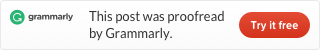Categories

# LeetCode 179 Largest Number [sort] java

Given a list of non negative integers, arrange them such that they form the largest number.

Example 1:

```Input: `[10,2]`
Output: "`210"````

Example 2:

```Input: `[3,30,34,5,9]`
Output: "`9534330"````

Note: The result may be very large, so you need to return a string instead of an integer.

In the first look, you may think just put the big number at first, like must put 9 before 3. But the input is not 1 digit number. So we know 5 smaller than 34, but 534 bigger than 345. Or 34 is smaller than 330, but 34330 bigger than 33034.

So when we compare two numbers, we can build two sorted results. For example, when we compare 34 and 330, we can add three 0 to 34 make to 34000+330, and another number is 330 add two 0, make to 33000+34.

Then we make this kind of compare function, like this:

nTen function to add 0 to number:：